Courses

# Data Interpretation MCQ -4

## 20 Questions MCQ Test General Aptitude for GATE 2020 | Data Interpretation MCQ -4

Description
This mock test of Data Interpretation MCQ -4 for Quant helps you for every Quant entrance exam. This contains 20 Multiple Choice Questions for Quant Data Interpretation MCQ -4 (mcq) to study with solutions a complete question bank. The solved questions answers in this Data Interpretation MCQ -4 quiz give you a good mix of easy questions and tough questions. Quant students definitely take this Data Interpretation MCQ -4 exercise for a better result in the exam. You can find other Data Interpretation MCQ -4 extra questions, long questions & short questions for Quant on EduRev as well by searching above.
QUESTION: 1

### Study the following graph carefully to answer the given questions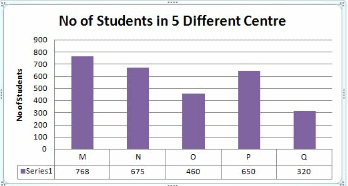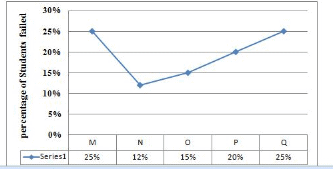If the ratio of number of boys & girls are in the ratio 3:2 in N and P, find the average number of boys in N and P together ?

Solution:

N:675 =>Boys = 675*3/5 = 405
P:650 =>Boys = 650*3/5 = 390
Avg = 405+390/2 = 397.5

QUESTION: 2

Solution:

M =768*25%= 192
O= 460*15% = 69
Q = 320*25% = 80
Total = 341

QUESTION: 3

### Study the following graph carefully to answer the given questionsWhat will be the ratio of number of boys passed in N to number of girls failed in N, if the number of girls and boys in the ratio 16:11 ?

Solution:

675 => 400:275 => 16:11 = G:B
G:400*12% =48
B:275*88% =242
242:48 = 121: 24

QUESTION: 4

Study the following graph carefully to answer the given questionsFind the difference between the number of passed students in Q to number of failed students in M ?

Solution:

Q passed => 75% of 320 = 240
M failed => 192
Difference = 240-192 = 48

QUESTION: 5

Study the following graph carefully to answer the given questionsWhich centre has the second least number of failed student ?

Solution:

M=192
N=81
O=69
P=130
Q=80

QUESTION: 6

Study the following table carefully to answer the given questions

Classification of 50 Students based on the marks obtained by them in Quants & Reasoning in Prelim exam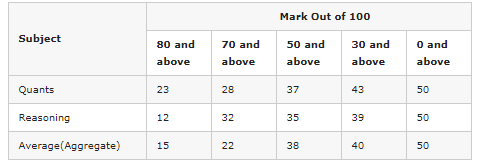The number of students scoring less than 30% marks in aggregate is?

Solution:

We have 30% of 100 = (30/100)x100 = 30
Therefore Required number
= Number of students scoring less than 30 marks in aggreagate
= 50 – Number of students scoring 30 and above marks in aggregate
= 50 – 40 = 10.

QUESTION: 7

Study the following table carefully to answer the given questions

Classification of 50 Students based on the marks obtained by them in Quants & Reasoning in Prelim examIf it is known that at least 20 students were eligible for main exam, then the minimum qualifying marks in Reasoning for eligibility to attend the main exam(Top 20 students only can attend main exam )?

Solution:

12 students secured above 80 then remaining 8 student will scored in the range 70-80

QUESTION: 8

Study the following table carefully to answer the given questions

Classification of 50 Students based on the marks obtained by them in Quants & Reasoning in Prelim examThe percentage of number of students scored 70% marks in Quants is what percentage of number of students scored 30% marks in aggregate ?

Solution:

Number of students scored 70% marks or 70 in Quants  = 28
Number of students scored 30% marks or 30 in aggregate= 40
% = 28/40 * 100 = 70%

QUESTION: 9

Study the following table carefully to answer the given questions

Classification of 50 Students based on the marks obtained by them in Quants & Reasoning in Prelim examIf at least 50% marks in aggregate are required for interview, how many students will be eligible to attend interview?

Solution:

50% of 100 = 50*100/100 = 50
No of students scored 50 aggregate = 38

QUESTION: 10

Study the following table carefully to answer the given questions

Classification of 50 Students based on the marks obtained by them in Quants & Reasoning in Prelim examWhat is the different between the number of students passed with 80 as cut-off marks in Quants and 30 as cut-off marks in aggregate together to no of students passed with 70 as cut-off marks in aggregate and 50 as cut off in Reasoning?

Solution:

Difference = (23+40 ) – ( 22+35) =6

QUESTION: 11

(Q.no: 11-15). Refer to the following Line Graph and answer the given questions.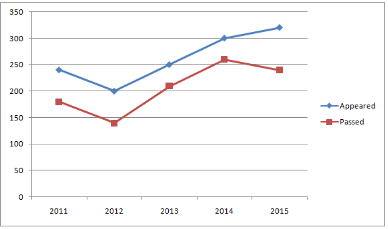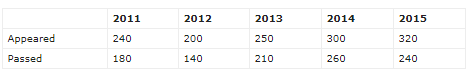The number of students who could pass the exam in the year 2016 were 10% more than that in the previous year. Only 75% of those who appeared in the year 2016 could pass the exam that year. How many students appeared for the exam in the year 2016?

Solution:

Number of students who could pass the exam in the year 2016 = 110% of 240 = 264
75% of x = 264
x = 264 * 100/75 = 352

QUESTION: 12

(Q.no: 11-15). Refer to the following Line Graph and answer the given questions.Number of students who had passed the exam in the year 2012 are what percent less than that in the year 2013?

Solution:

Required % = (70/210) * 100 = 100/3 %

QUESTION: 13

(Q.no: 11-15). Refer to the following Line Graph and answer the given questions.What percent of students who appeared for the exam in the year 2011 could pass the exam?

Solution:

% = 180/240 * 100 = 75%

QUESTION: 14

(Q.no: 11-15). Refer to the following Line Graph and answer the given questions.What is the respective ratio of total number of students who appeared for the exam in the years 2014 and 2015 together and the total number of students who could pass the exam in the same years together?

Solution:

Total number of appeared candidates in the years 2014 and 2015 together = 620
Total number of passed candidates in the years 2014 and 2015 together = 520
= 620 : 500
= 31 : 25

QUESTION: 15

(Q.no: 11-15). Refer to the following Line Graph and answer the given questions.What is the average number of students who passed for the exam in all the years together?

Solution:

Average number of students who passed in all the years together =[180 + 140 + 210 + 260 +240]/5 = 206

QUESTION: 16

(Q.no: 16-20). Refer to the following table and answer the given questions.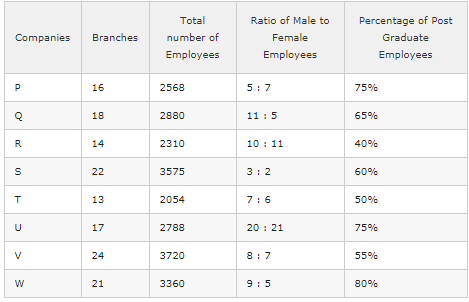If the number of male post graduate employees in company W is 1488, what percent of the female employees in that particular company are post graduate?

Solution:

Number of Females in Company W = 3360 *(5/14) = 1200
Number of Post graduate employees in Company W = 3360 *(80/100) = 2688
Number of female post graduate employees in Company W = 2688 – 1488 = 1200
Required Percentage = (1200 * 100)/1200 = 100%

QUESTION: 17

(Q.no: 16-20). Refer to the following table and answer the given questions.In which of the given companies is the percentage of women employees with respect to the total number of employees(both males and females) in that company the third lowest?

Solution:

Company P : ([7/12 * (2568)] / 2568)*100 = 58.33%
Company Q : ([5/16 * (2880)] / 2880)*100 = 31.25%
Company R : ([11/21 * (2310)] / 2310)*100 = 52.38%
Company S : ([2/5 * (3575)] / 3575)*100 = 40%
Company T : ([6/13 * (2054)] / 2054)*100 = 46.15%
Company U : ([21/41 * (2788)] / 2788)*100 = 51.21%
Company V : ([7/15 * (3720)] / 3720)*100 = 46.66%
Company W : ([5/14 * (3360)] / 3360)*100 = 35.71%

QUESTION: 18

(Q.no: 16-20). Refer to the following table and answer the given questions.What is the ratio of the total number of male employees in companies Q and W together to the total number of female employees in Companies R and S together?

Solution:

= [2880 * (11/16)/ 2310 * (11/21) + 3360 * (9/14)/ 3575 * (2/5)] = (1980 + 2160)/(1210 + 1430) = 414/264 = 69:44

QUESTION: 19

(Q.no: 16-20). Refer to the following table and answer the given questions.What is the sum of the average number of post graduate employees in companies P, Q and S together and the average number of post graduate employees in companies U, V and W?

Solution:

Average number of post graduate employees in companies P, Q and S = (1926 + 1872 + 2145)/3 = 5943/3 = 1981
Average number of post graduate employees in companies U, V and W = (2091 + 2046 + 2688)/3 = 6875/3 = 2275
Difference = 2275 + 1981 = 4256

QUESTION: 20

(Q.no: 16-20). Refer to the following table and answer the given questions.Which of the given companies has the second highest number of average employees per office?

Solution:

Company P – 2568/16 = 160.5 ; Company Q – 2880/18 = 160
Company R – 2310/14 = 165 ; Company S – 3575/22 = 162.5
Company T – 2054/13 = 158 ; Company U – 2788/17 = 164
Company V – 3720/24 = 155 ; Company W – 3360/21 = 160